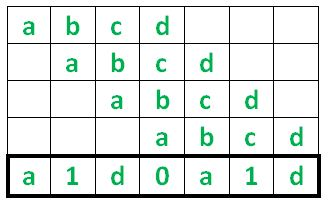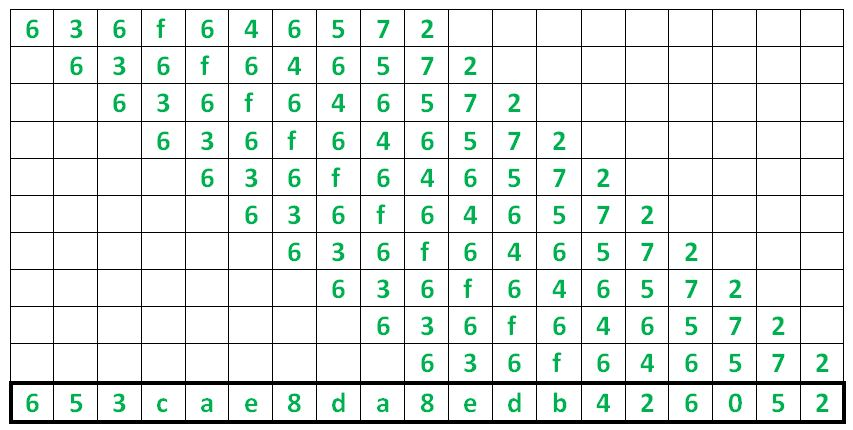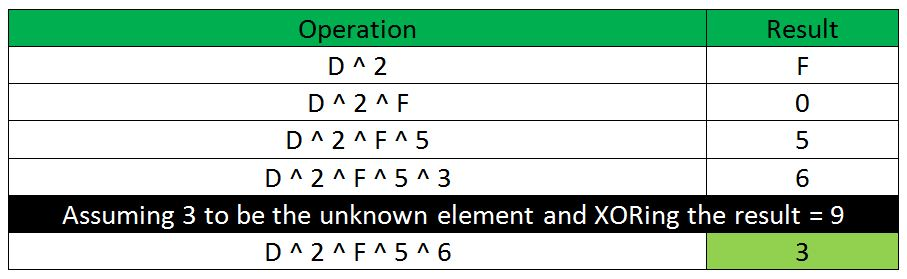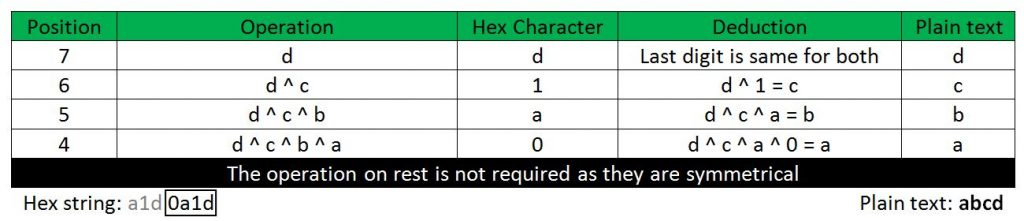Open in App
Not now

# XOR Encryption by Shifting Plaintext

• Difficulty Level : Hard
• Last Updated : 21 Jul, 2022

Here is a cipher algorithm, based on hexadecimal strings that is implemented by XORing the given plaintext, N number of times where N is its length. But, the catch is that every next XOR operation is done after shifting the consecutive plain text entry to the right. A sample operation is shown below :Suppose the password is ‘abcd’ then the hexadecimal text is calculated as a1d0a1d by XORing the password with itself N times i.e. 4 times in this case. Similarly if the password is ‘636f646572’, then653cae8da8edb426052 is the hexadecimal text. So, the problem statement is to create a decryption algorithm (in any programming language) and deduce the plain text from the given hexadecimal string. Examples :

```Input : a1d0a1d
Output : abcd
abcd once coded will return a1d0a1d

Input : 653cae8da8edb426052
Output : 636f646572```

Approach : The key ingredient in encrypting and decrypting is in the properties of XOR. XOR is a bitwise operation where the result is 0 if the two possible inputs are same but 1 when the inputs are different. The XOR table is given below for reference :

An important and useful property of XOR that is widely popular in cryptography is that in case of multiple XORing of numbers (say M numbers), if we know only the M – 1 numbers (one is unknown) along with the XOR result then, we can easily calculate the missing number by XORing the known numbers and the XOR result. This property is discussed with the following hexadecimal numbers :We shall be using the above listed property the most in course of this problem. Now, if we look at the encryption diagram of ‘abcd’ at the base it is just the repeated XORing of the digits. The rightmost digit is d and the rightmost digit of the ‘abcd’ is d as well so the last digit of both plaintext and hexstring is the same. The next digit is 1 which is calculated by XORing the second right digit of abcd and the previous digit i.e. 1 = d ^ c using the property we know the plain text digit can be deduced as d ^ 1 = c. Similarly, the next digit is a which is found by d ^ c ^ b = a. We only need to do this till the half of the hex string as the rest is symmetrical so they are not required.Below is the implementation of above approach :

## C++

 `// Implementation of the code in C++` `#include ` `using` `namespace` `std;`   `int` `main()` `{`   `  ``// Hex String variable` `  ``string hex_s = ``"653cae8da8edb426052"``;`   `  ``// Plain text variable` `  ``string plain = ``""``;`   `  ``// variable to store the XOR` `  ``// of previous digits` `  ``int` `x = 0;`   `  ``int` `l = hex_s.length();`   `  ``// Loop for loop from the end to` `  ``// the mid section of the string` `  ``for` `(``int` `i = l - 1; i > (l / 2) - 1; i--) {` `    ``string digit = ``""``;` `    ``digit += hex_s[i];`   `    ``// calculation of the plaintext digit` `    ``unsigned ``int` `y = x ^ stoul(digit, nullptr, 16);`   `    ``// calculation of XOR chain` `    ``x = x ^ y;` `    ``stringstream sstream;` `    ``sstream << hex << y;` `    ``string z = sstream.str();` `    ``plain = z[z.length() - 1] + plain;` `  ``}`   `  ``cout << plain;` `}`   `// This code is contributed by phasing17`

## Java

 `// Implementation of the code in Java`     `class` `GFG {` `    ``public` `static` `void` `main(String[] args) {` `        ``// Hex String variable` `        ``String hex_s = ``"653cae8da8edb426052"``;` `        `  `        ``// Plain text variable` `        ``String plain = ``""``;` `        `  `        ``// variable to store the XOR` `        ``// of previous digits` `        ``int` `x = ``0``;` `        `  `        ``int` `l = hex_s.length();` `        `  `        ``// Loop for loop from the end to` `        ``// the mid section of the string` `        ``for` `(``int` `i = l - ``1``; i > (l / ``2``) - ``1``; i--)` `        ``{` `            ``// calculation of the plaintext digit` `            ``int` `y = x ^ Integer.parseInt(Character.toString(hex_s.charAt(i)), ``16``);` `            `  `            ``// calculation of XOR chain` `            ``x = x ^ y; ` `            ``String z = Integer.toString(y, ``16``);` `            ``plain = z.charAt(z.length() - ``1``) + plain;` `        ``}  ` `        `  `        ``System.out.println(plain);` ` `  `    ``}` `}`   `//This code is contributed by phasing17`

## Python

 `# Implementation in Python 3`   `# Hex String variable` `hex_s ``=` `'653cae8da8edb426052'`   `# Plain text variable` `plain ``=` `''`   `# variable to store the XOR` `# of previous digits` `x ``=` `0`   `l ``=` `len``(hex_s)`   `# Loop for loop from the end to` `# the mid section of the string` `for` `i ``in` `range``(l ``-` `1``, ``int``(l ``/` `2``) ``-` `1``, ``-``1``):` `    `  `    ``# calculation of the plaintext digit` `    ``y ``=` `x^``int``(hex_s[i], ``16``)` `    `  `    ``# calculation of XOR chain` `    ``x ``=` `x^y` `    ``plain ``=` `hex``(y)[``-``1``] ``+` `plain` `    `  `print``(plain)`

## C#

 `// Implementation of the code in C#` `using` `System;`   `class` `GFG {`   `  ``public` `static` `void` `Main(``string``[] args)` `  ``{`   `    ``// Hex String variable` `    ``string` `hex_s = ``"653cae8da8edb426052"``;`   `    ``// Plain text variable` `    ``string` `plain = ``""``;`   `    ``// variable to store the XOR` `    ``// of previous digits` `    ``int` `x = 0;`   `    ``int` `l = hex_s.Length;`   `    ``// Loop for loop from the end to` `    ``// the mid section of the string` `    ``for` `(``int` `i = l - 1; i > (l / 2) - 1; i--) {` `      ``string` `digit = ``""``;` `      ``digit += hex_s[i];`   `      ``// calculation of the plaintext digit` `      ``int` `y = x ^ Convert.ToInt32(digit, 16);`   `      ``// calculation of XOR chain` `      ``x = x ^ y;` `      ``string` `z = Convert.ToString(y, 16);` `      ``plain = z[z.Length - 1] + plain;` `    ``}`   `    ``Console.WriteLine(plain);` `  ``}` `}`   `// This code is contributed by phasing17`

## Javascript

 `// Implementation of the code in JS`   `// Hex String variable` `var` `hex_s = ``'653cae8da8edb426052'``;`   `// Plain text variable` `var` `plain = ``''``;`   `// variable to store the XOR` `// of previous digits` `var` `x = 0;`   `var` `l = hex_s.length;`   `// Loop for loop from the end to` `// the mid section of the string` `for` `(``var` `i = l - 1; i > (l / 2) - 1; i--)` `{` `    ``// calculation of the plaintext digit` `    ``var` `y = x ^ parseInt(hex_s[i], 16);` `    `  `    ``// calculation of XOR chain` `    ``x = x^y;l` `    ``plain = y.toString(16).slice(-1) + plain;` `}  `   `console.log(plain);`   `// this code is contributed by phasing17`

Output:

`636f646572`

Time Complexity : O(l) ,where l is size of hex string

Auxiliary Space : O(1)

My Personal Notes arrow_drop_up
Related Articles